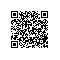# 独家 | 手把手教你用Python构建你的第一个多标签图像分类模型（附案例）

## 介绍

• 对象检测
• 图像分割
• 图像翻译
• 对象跟踪(实时)，还有更多……

https://www.analyticsvidhya.com/blog/2019/01/build-image-classification-model-10-minutes/?utm_source=blog&utm_medium=multi-label-image-classification## 目录

1. 什么是多标签图像分类？
2. 多标签图像分类与多类图像分类有何不同？
3. 了解多标签图像分类模型体系结构；
4. 构建多标签图像分类模型的步骤；
5. 案例研究：用Python解决多标签图像分类问题；

6.接下来的步骤和你的实验；

7.尾记。

## 1. 什么是多标签图像分类？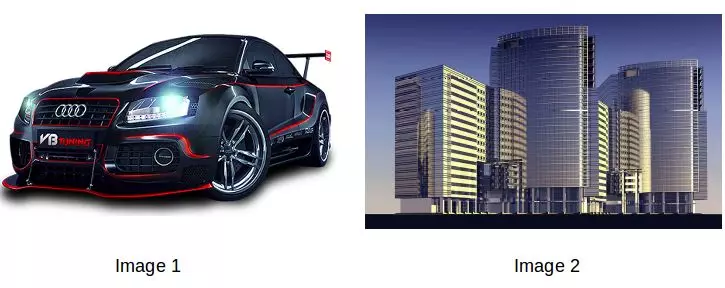## 2. 多标签图像分类与多类图像分类有何不同？

• 每个图像只包含一个对象(上述4个类别中的任何一个)，因此，它只能被归入4个类别中的一个。
• 图像可能包含多个对象(来自上述4个类别)，因此该图像将属于多个类别。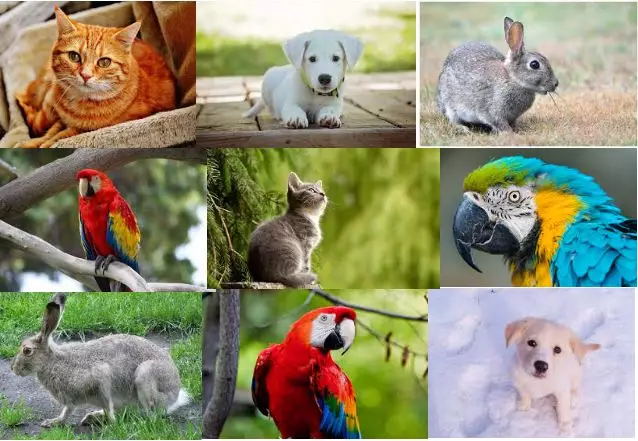• 当图像可分类的类别超过两种时
• 一个图像不属于一个以上的类别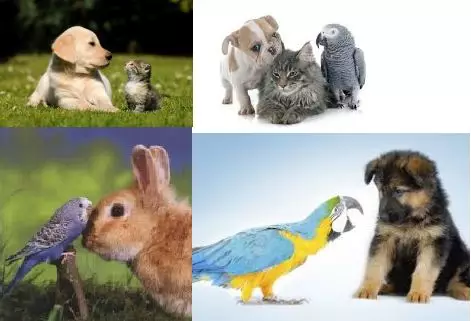• 第一张图片(左上角)包含一只狗和一只猫
• 第二幅图(右上角)包括一只狗、一只猫和一只鹦鹉
• 第三幅图(左下角)包含一只兔子和一只鹦鹉，以及
• 最后一张图片(右下角)包含一只狗和一只鹦鹉

https://www.analyticsvidhya.com/blog/2019/01/build-image-classification-model-10-minutes/?utm_source=blog&utm_medium=multi-label-image-classification

## 5. 案例研究：用Python解决多标签图像分类问题https://www.cs.ccu.edu.tw/~wtchu/projects/MoviePoster/index.html

1. import keras
2. from keras.models import Sequential
3. from keras.layers import Dense, Dropout, Flatten
4. from keras.layers import Conv2D, MaxPooling2D
5. from keras.utils import to_categorical
6. from keras.preprocessing import image
7. import numpy as np
8. import pandas as pd
9. import matplotlib.pyplot as plt
10. from sklearn.model_selection import train_test_split
11. from tqdm import tqdm
12. %matplotlib inline

2. train.head() # printing first five rows of the file1. train.columns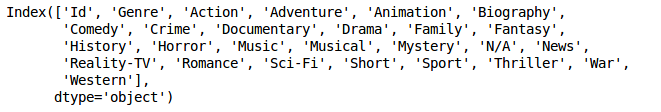Genre列包含每个图像的列表，其中明确了每个图像对应的电影的类型。因此，从.csv文件的头部开始，第一个图像的类型是喜剧和戏剧。

1. train_image = []
2. for i in tqdm(range(train.shape)):
4. img = image.img_to_array(img)
5. img = img/255
6. train_image.append(img)
7. X = np.array(train_image)

1. X.shape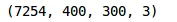1. plt.imshow(X)1. train'Genre'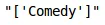1. y = np.array(train.drop(['Id', 'Genre'],axis=1))
2. y.shape

1. X_train, X_test, y_train, y_test =
2. train_test_split(X, y, random_state=42, test_size=0.1)

1. model = Sequential()
2. model.add(Conv2D(filters=16, kernel_size=(5, 5), activation="relu", input_shape=(400,400,3)))

1. model.summary()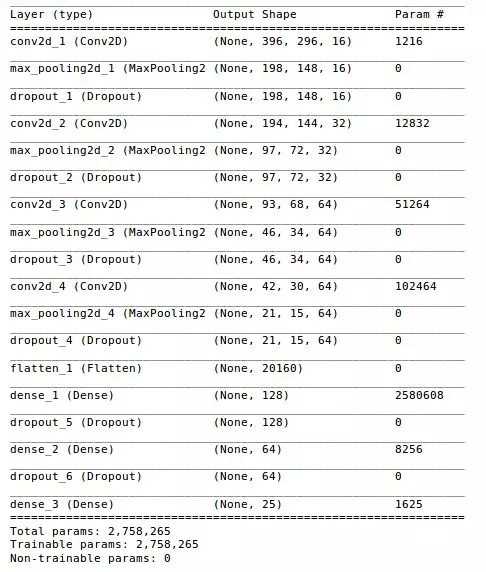1. model.fit(X_train, y_train, epochs=10, validation_data=(X_test, y_test), batch_size=64)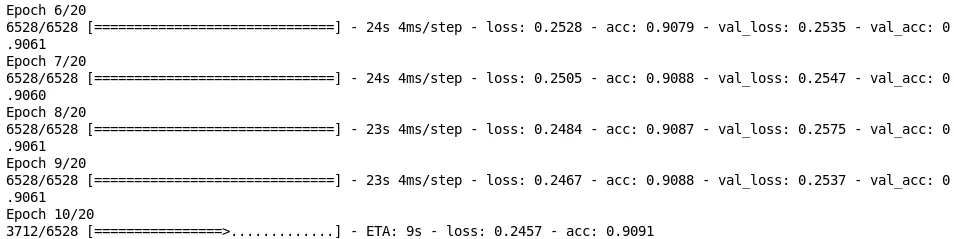GOT

Avengers

2. img = image.img_to_array(img)
3. img = img/255

1. classes = np.array(train.columns[2:])
2. proba = model.predict(img.reshape(1,400,400,3))
3. top_3 = np.argsort(proba)[:-4:-1]
4. for i in range(3):
5. print("{}".format(classes[top_3[i]])+" ({:.3})".format(proba0]))
6. plt.imshow(img)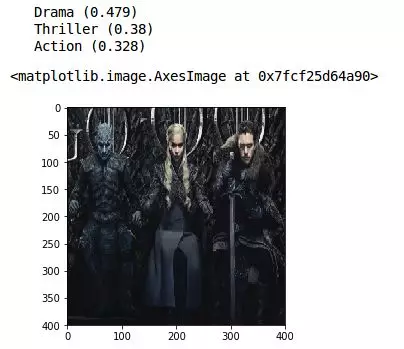2. img = image.img_to_array(img)
3. img = img/255

1. classes = np.array(train.columns[2:])
2. proba = model.predict(img.reshape(1,400,400,3))
3. top_3 = np.argsort(proba)[:-4:-1]
4. for i in range(3):
5. print("{}".format(classes[top_3[i]])+" ({:.3})".format(proba0]))
6. plt.imshow(img)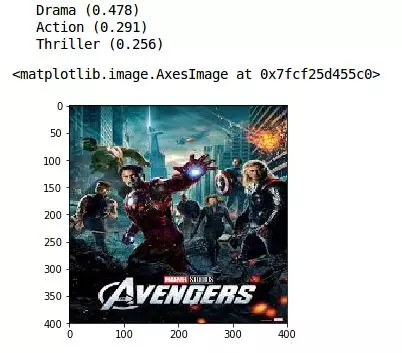2. img = image.img_to_array(img)
3. img = img/255

1. classes = np.array(train.columns[2:])
2. proba = model.predict(img.reshape(1,400,400,3))
3. top_3 = np.argsort(proba)[:-4:-1]
4. for i in range(3):
5. print("{}".format(classes[top_3[i]])+" ({:.3})".format(proba0]))
6. plt.imshow(img)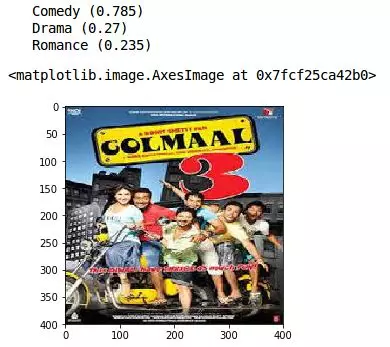《Golmaal 3》是一部喜剧，我们的模型预测它为最受欢迎的类型。其他预测类型是剧情片和浪漫片——相对准确的评估。我们可以看到该模型能够仅通过海报预测电影类型。

## 7. 尾记

https://www.analyticsvidhya.com/blog/2019/04/build-first-multi-label-image-classification-model-python/

Build your First Multi-Label Image Classification Model in Python

### 译者简介